Vibroengineering PROCEDIA

Published: 18 May 2023

# Optimization of process parameters for acid fracturing assisted herringbone well SAGD

Xi Yi1
Guangsheng Cao2
Jiaqi Tang3
1, 2, 3Northeast Petroleum University, Key Laboratory of Enhanced Oil and Gas Recovery, Ministry of Education, Daqing, Heilongjiang, 163318, China
Corresponding Author:
Guangsheng Cao
Views 8

#### Abstract

Zhong18 wellblock of Fengcheng oilfield developed with low permeability interlayer and had strong heterogeneity, which caused steam chamber of conventional steam assisted gravity drainage (SAGD) process cannot extend lengthwise continuously and serious steam heat loss. To address this problem, a method of acid fracturing assisted SAGD process is proposed to enhance the oil drainage and improve oil recovery. Based on the herringbone well SAGD being used in Zhong18 wellblock, the orthogonal experimental design and oil reservoir numerical simulation are used to determine the significance level and fracture parameter optimization of the acid fracturing process. The priority of optimization sequence is: fracture permeability, fracture width, fracture half-length, fracture spacing. The optimal parameters are as: 3D fracture permeability, 2.8 mm fracture width, 80 m fracture half-length and 80 m fracture spacing. A 10-year simulation using optimized parameters can improve the recovery by 9.2 % compared with the conventional SAGD process. The result proved that acid fracturing assisted herringbone well SAGD process can effectively penetrate the low permeability interlayer, expand the sweep range of steam chamber, and improve the oil recovery degree of the reservoir.

## 1. Introduction

In 2015, Fengcheng oilfield in Xinjiang, China, took the lead in combining SAGD development technology with herringbone horizontal well [1-2]. Herringbone well SAGD is an extension of the traditional dual horizontal well SAGD, where the horizontal injection well is replaced by a herringbone horizontal well, with branches sloping upward along the horizontal well section, and spread into herringbone shape (as shown in Fig. 1). Through branching deep into the reservoir, the steam injection capacity can be increased at the same injection pressure, and the drainage range of steam chamber can also be increased.

Fig. 1Sketch map of the herringbone well SAGD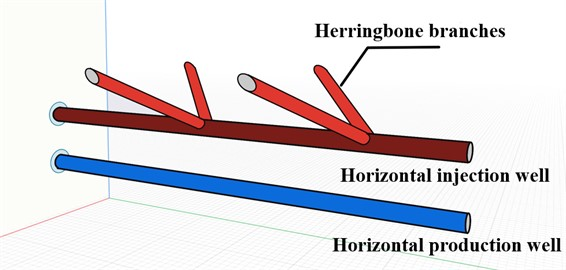The J3q3 formation in the Zhong 18 well block was tested for SAGD development in 2012. Up to now, 96 well sets have been put into SAGD production, with an average production time of 5 years. The average daily steam injection per well is 57.0t/d; the average daily oil production per well is 8.2t/d; the oil-vapor ratio is 0.16, and the recovery degree is 10.8 %. The development effect is not satisfied. The formation and expansion of steam chamber is the key of steam-assisted gravity drainage (SAGD) technology. However, during actual field test, steam chambers are difficult to develop well due to the strong heterogeneity of the reservoir. Because of the low permeability of interlayers, the vertical expansion of steam is blocked, also the steam heat loss is serious, these reasons led to the overall production effect in the early stage is not ideal. In the application of oilfield development, the acid fracturing technology can overcome the difficulties of reservoirs with strong heterogeneity and low permeability interlayer [3-4]. To this end, an acid fracturing assisted SAGD stimulation technology is proposed to enhance oil drainage. Acid fracturing can replace the proppant used in other fracturing methods . Its working principle refers to the high-pressure injection of acid fracturing working fluid into the oil and gas reservoir, by pressurizing the formation to create long hydraulic fractures, and meanwhile fully contact with the heterogeneous rock layer and produce a series of comprehensive reactions with the alkaline substances in the rock layer, forming acid etching fractures in the form of earthworms while obtaining a good pollution release effect in the near-well zone [6-7]. How to improve the flow conductivity of acid etching fractures is the key to evaluate the acid fracturing technology in oilfield [8-9]. Based on the conventional acid fracturing working fluid of sand stone reservoirs [10-11], considering the need for corrosion inhibition in the high temperature working environment of SAGD process, as well as the extremely acid sensitive characteristics of the formation in the Zhong18 wellblock, the acid formulation of the study block is set to 2% hydrofluoric acid + 3 % fluoroboric acid + 6 % hydrochloric acid. The main factors affecting the conductivity of acid etching fractures are fracture permeability, fracture width, fracture half-length and fracture spacing. The best solution to the above factors is of great significance for the implementation of acid fracturing technology in target blocks. The oil reservoir numerical simulation method is used to optimize the increment of recovery degree of different schemes. The optimization results will provide certain guidance for the actual acid fracturing stimulation in the field.

## 2. Dynamic fracture propagation model

Based on the plane strain, a two-dimensional PKN model is used to solve the fracture extension along the length direction, and another two-dimensional KGD model is used to solve the growth of fracture height . Assuming that the fracture length is longer than the fracture height, a dynamic proposed 3D model of fracture extension is established. According to this model, the vertical component of the acid is less important than the radial component, that is, the dynamic fracture height depends on the position and time of the fracture length.

### 2.1. Dynamic fracture width equation

1
$W\left(x,y\right)=\frac{8\left(1-{v}^{2}\right)h\left(x\right)}{\pi E}{\int }_{{\eta }_{f}}^{1}\frac{\tau d\tau }{\sqrt{{\tau }^{2}-{{\eta }_{f}}^{2}}}{\int }_{0}^{\tau }\frac{p\left(y\right)dy}{\sqrt{{\tau }^{2}-{y}^{2}}},$

where ${\eta }_{f}=\frac{2y}{h\left(x\right)}$; $W\left(x,y\right)$ represents the fracture width at the fracture length $x$ and the fracture height $y$ at time $t$, m; $v$ stands for the Poisson ratio, dimensionless; $E$ represents the modulus of elasticity, MPa; $P\left(y\right)$ represents the pressure at the fracture height $y$, MPa.

### 2.2. Dynamic fracture height equation

2
$-\frac{\partial p}{\partial x}=\frac{1}{h}\left(\frac{{k}_{c}}{2\sqrt{\pi {h}_{\frac{1}{2}}}}-\frac{2f\mathrm{\Delta }s}{\pi \sqrt{1-{f}^{2}}}\right)\frac{d{h}_{\frac{1}{2}}}{dx},$

where ${k}_{c}$ stands for stress intensity factor, MPa·m1/2; ${h}_{1/2}$ represents the half-height of the fracture, m; $\mathrm{\Delta }s$ means the stress difference, MPa; $f$ is the ratio of reservoir thickness to the fracture height, dimensionless.

### 2.3. Mathematical model of acid dosage

3
$Q=\frac{2W{L}_{fe}h\left(1-\varphi \right)}{Nt},$

where $Q$ represents the acid fluid displacement, m3/min; ${L}_{fe}$ represents the effective action distance of acid solution, m;$N$ indicates the dissolution capacity of the acid solution, dimensionless.

## 3. Numerical model

Fengcheng Oilfield is located in the northwest edge of Junggar basin. The reservoir lithology of Zhong18 wellblock is mainly medium fine sand, and the reservoir is characterized by strong acid sensitivity. SAGD development zone is a monoclinic reservoir controlled lithologic, located in pure oil area, belongs to a shallow ultra-heavy oil reservoir. The Builder module of CMG reservoir numerical simulation software is selected, the model grid step is set to 10 m×10 m; the number of grids is divided into 72×43 (3,096) on the plane; the number of small layers is finely divided into 26 on vertical, and the total number of grids is 72×43×26 (80,496). Fig. 2 and Fig. 3 are the diagrams of numerical simulation model.

Fig. 2The diagram of numerical simulation model of structure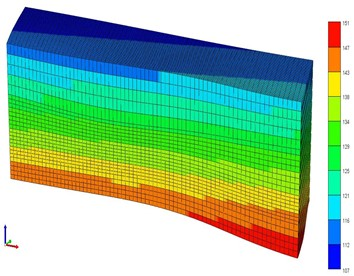Fig. 3The diagram of numerical simulation model of oil saturation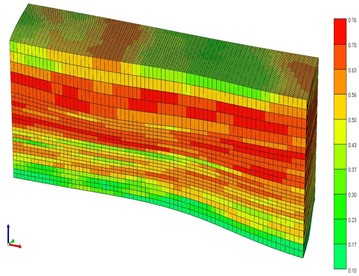Based on reservoir characteristics, the numerical model of SAGD well group is established to complete the historical fitting. The main parameters of the model include: the average reservoir depth is 460 m; the average reservoir thickness is 19.5 m; the reservoir temperature is 25 ℃; the reservoir pressure is 4.3 MPa; the reservoir average permeability is 783 mD; the vertical to horizontal permeability ratio is 0.6; the average porosity is 28 %; the average oil saturation is 65 %; the crude oil density is 0.966 g/cm3, and the viscosity of degassed crude oil is 114970 mPa·s at 50 ℃. The fracture width of the dynamic fracture propagation model is calculated by Eq. (1), and the fracture height of the model is calculated by Eq. (2). The acid dosage is calculated by Eq. (3).

## 4. Fracture parameter optimization

### 4.1. Fracture width optimization

The fracture permeability is the key to form fractures. According to the practical engineering, the fracture permeability can be controlled at about 3D by the existing acid fracturing technology. Therefore, the fracture permeability is selected as 3D, the simulated production time is 48 months, and the fracture width, fracture half-length and fracture spacing are optimized sequentially.

The fracture width produced by dissolution increases with the increase of acid injection volume and acid injection displacement, and the fracture width is a controllable engineering factor . The fracture width is set between 2.0 mm and 3.8 mm, with a variable range of every 2 mm. It can be seen from Fig. 4 that the degree of oil recovery gradually increases as the fracture width increases. When the fracture width reaches 2.4 mm, the recovery degree increases sharply. When the fracture width continues to expand to 2.8 mm, the increase in the degree of recovery peaks. Expanding the fracture width can significantly improve the flow conductivity of the fracture and further enhance the permeability of the reservoir, and the cost of creating the fracture also increases with it. The optimal width of the fracture is 2.8 mm.

Fig. 4Variation of recovery degree with different fractures widths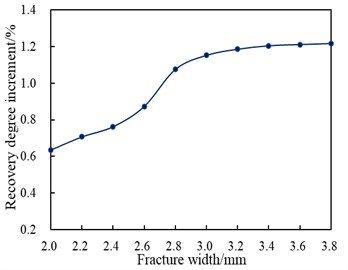Fig. 5Variation of recovery degree with different facture half-lengths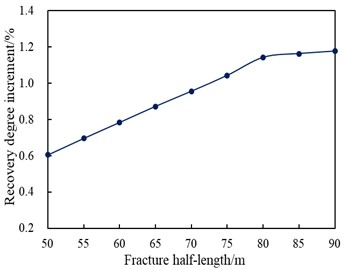### 4.2. Fracture half-length optimization

The fracture half-length produced by acid fracturing is determined by both the inflow distance into the fracture before acid depletion and the acid etching on the fracture surface, and the fracture half-length is a controllable parameter . The fracture half-length is set between 50 and 90 m, with a variable range of every 5 m. It can be seen from Fig. 5, the increment of recovery degree is positively correlated with the fracture half-length. When the fracture half-length reaches 80 m, the increase of recovery degree reaches the peak. The fracture half-length increases gradually after 80 m. The fracture half-length can effectively control the sweep range of the acid fracturing process. The longer the fracture half-length, the wider the sweep ranges. Whereas extending the fracture half-length will make the construction more difficult, so the optimal fracture half-length should be 80 m.

### 4.3. Fracture spacing optimization

Proper drainage distance between fractures can give full play to the high inflow capacity of acid-etching fractures, which is conducive to improving the permeability of the reservoir . To screen the optimal fracture spacing, the fracture spacing is set between 40 and 100 m, with a variable range of every 10 m. Fig. 6(a) shows the effect of the fracture spacing on recovery degree.

Fig. 6a) Stimulation at different fracture spacing, b) Variation of recovery degree with different fracture spacings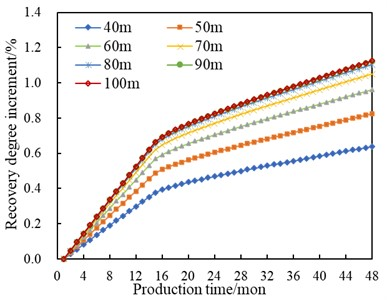a)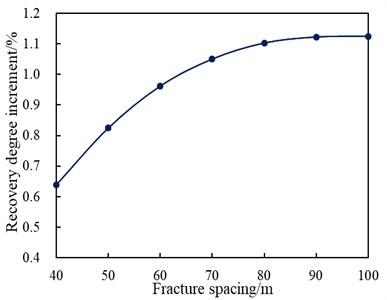b)

It can be seen from Fig. 6(b) that the increment of recovery degree varies with the increase of fracture spacing in a smooth curve. When the fracture spacing reaches 80 m, the curve tends to be gentle with the increase of the variable path. Fracture spacing is affected by the fracture permeability, fracture width and fracture half-length. When the distance is too small, the fractures interfere with each other, which is not conducive to the development. When the distance increases to a certain value, the flow-conductivity of each fracture can be given full play and the significance of continuing to increase the distance to increase production is not obvious. The optimal fracture spacing is 80 m.

Using the numerical model of acid fracturing in herringbone well SAGD, the optimized fracture parameters are used: fracture width of 2.8 mm, fracture half-length of 80 m and fracture spacing of 80 m. Based on the 10-year production time and process simulation, the accumulated oil increase is 3.2×104m3, the average water content is 11 %; the average daily oil production is increased by 8.8 m3/d, and the recovery degree can reach 57.5 %. Compare to un-optimized simulation, the recovery degree in each zone has improved, with an average increase of 9.2 %. The optimization strategy of acid fracturing parameters has certain reference significance for the actual acid fracturing stimulation on site.

## 5. Conclusions

1) The dynamic expansion model of fracture height and fracture length, and the calculation equation of acid dosage are given for SAGD acid fracturing in sandstone formation.

2) After orthogonal parameter design and reservoir numerical simulation study, the best combination of fracture parameters for acid fracturing assisted herringbone well SAGD process in the Zhong18 wellblock of Fengcheng oilfield is: 3D fracture permeability, 2.8 mm fracture width, 80m fracture half-length and 80 m fracture spacing.

3) The smaller the volume sweep coefficient before parameter optimization, the higher the value of volume sweep coefficient improvement after optimization. The volume sweep coefficient and the sweep range of steam chamber are greatly improved. The recovery degree of 10 years simulated production with optimized fracture parameters is 9.2 % higher than un-optimized simulation.

#### Acknowledgements

The authors have not disclosed any funding.